﻿ 湖南省经济活力的预测及其影响因素的研究 The Prediction of Economic Vitality in Hunan Province and Its Influencing Factors

Statistics and Application
Vol. 09  No. 01 ( 2020 ), Article ID: 34114 , 9 pages
10.12677/SA.2020.91010

The Prediction of Economic Vitality in Hunan Province and Its Influencing Factors

Jian Luo, Xin Zhang, Yihang Li

College of Information and Intelligent Science and Technology, Hunan Agricultural University, Changsha Hunan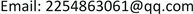Received: Jan. 17th, 2020; accepted: Jan. 31st, 2020; published: Feb. 6th, 2020ABSTRACT

In order to comprehensively study the economic vitality and its influencing factors of Hunan Province, this paper, on the one hand, discusses from two specific perspectives of population and enterprise, considers the relevant indicators from an objective level, establishes a quantitative relationship model to analyze its influencing factors, and reveals the endogenous influencing factors of economic vitality; on the other hand, starts from the macro-control of the government, and considers Hunan Province in 2016. The implementation of supply side structural reform defines economic vitality factors from two aspects of economy and environment, and establishes a short-term prediction model of economic vitality based on time series to reveal the subjective impact of government policies on economic vitality. Finally, some suggestions are given for the economic development of Hunan Province.

Keywords:Economic Vitality, Government Policy, Econometric Relationship, Time Series Prediction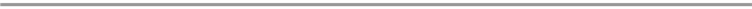1. 引言

2. 数据来源与模型假设

1) 搜寻的数据真实可靠。

2) 选取的每个解释变量都是独立的。

3) 人均GDP的一阶差分序列是统计平稳的。

3. 基于人口与企业视角下的计量关系模型

3.1. 问题的分析与模型的选择

3.2. 变量的确立

3.2.1. 被解释变量的确立Table 1. Gross regional product of Hunan province (one hundred million yuan)

3.2.2. 关键解释变量的确立Table 3. Number of legal entities in Hunan Province (one)

3.2.3. 其它解释变量的确立

3.3. 计量关系模型的建立

3.3.1. 数据比值归一化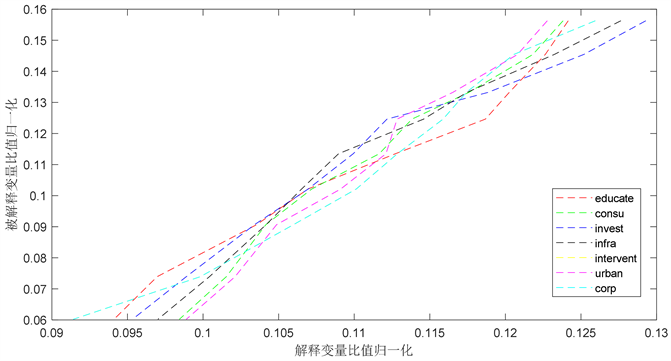Figure 1. Functional diagram

3.3.2. 模型的建立

$Y=f\left(urban,corp,educate,consu,invest,\mathrm{inf}ra,\mathrm{int}ervent\right)$ (1)

$\begin{array}{l}\mathrm{Re}GDP={\beta }_{0}+{\beta }_{1}urban+{\beta }_{2}corp+{\beta }_{3}educate+{\beta }_{4}consu+\\ \text{\hspace{0.17em}}\text{\hspace{0.17em}}\text{\hspace{0.17em}}\text{\hspace{0.17em}}\text{\hspace{0.17em}}\text{\hspace{0.17em}}\text{\hspace{0.17em}}\text{\hspace{0.17em}}\text{\hspace{0.17em}}\text{\hspace{0.17em}}\text{\hspace{0.17em}}\text{\hspace{0.17em}}\text{\hspace{0.17em}}\text{\hspace{0.17em}}{\beta }_{5}invest+{\beta }_{6}\mathrm{inf}ra+{\beta }_{7}\mathrm{int}ervent+\epsilon \end{array}$(2)

3.4. 计量关系模型的求解Table 6. Regression results of Econometric Relationship Model

1) 城市化水平系数显著为正，说明城镇人口比例的增加有利于促进湖南省经济的发展，它们之间具有较强的正相关性。事实上，城镇是第二、三产业发展的集中区域，是一个地区经济发展的领头羊。因此，从人口视角来看，湖南省的经济活力依赖于城市化水平的提高，依赖于城镇人口比例的增加。另外，从城镇人口比例以递增的趋势逐年增加可以得知，近年来湖南省的经济活力取得了较大的提高。

2) 企业数量系数为负，说明企业数量的增加相对其它变量对经济活力的促进作用较弱，原因可能是近年来随着创业的火热，企业数量增长较快，然而实际GDP增长速度远不如此，所以二者的相关性较弱，甚至在一定程度上会阻碍GDP的增长。

3) 对于其它解释变量，也可解释如下。受教育程度水平的系数为正，说明高等教育的水平可以显著影响经济活力，事实上，高等教育越发达，一个地区的人力资源，生产力的优势越大，因而经济活力越强。居民消费水平和基础实施建设的系数也为正且大于1，说明它们也是经济活力的主要正向影响因素。

4. 基于时间序列的短期经济活力预测模型

4.1. 政策实施效果评价模型

${\eta }_{1}=\frac{{\text{GDP}}_{第一产业}}{总\text{GDP}},{\eta }_{2}=\frac{{\text{GDP}}_{第二产业}}{总\text{GDP}},{\eta }_{3}=\frac{{\text{GDP}}_{第三产业}}{总\text{GDP}}$ (3)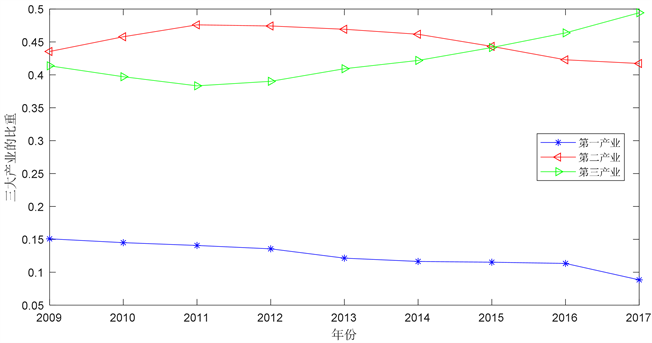Figure 2. Sequence chart of proportion of three industries

4.2. 短期经济活力预测模型

4.2.1. 湖南省经济活力因子的定义

$\mu =\frac{人均\text{GDP}}{I}$ (4)

4.2.2. 基于一阶差分指数平滑法的人均GDP预测模型

$\nabla {y}_{t}={y}_{t}-{y}_{t-1}$ (5)

$\nabla {\stackrel{^}{y}}_{t+1}=\alpha \nabla {y}_{t}+\left(1-\alpha \right)\nabla {\stackrel{^}{y}}_{t}$ (6)

${\stackrel{^}{y}}_{t+1}=\nabla {\stackrel{^}{y}}_{t+1}+{y}_{t}$ (7)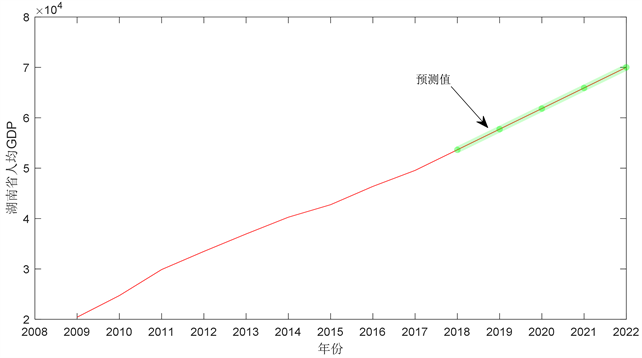Figure 3. Time series chart of Hunan Province’s per capita GDP forecast

4.2.3. 基于移动平均法的工业污染治理投资预测模型

$\begin{array}{l}{M}^{\left(1\right)}=\frac{1}{N}\left({y}_{t}+{y}_{t-1}+\cdots +{y}_{t-N+1}\right)\\ \text{\hspace{0.17em}}\text{\hspace{0.17em}}\text{\hspace{0.17em}}\text{\hspace{0.17em}}\text{\hspace{0.17em}}\text{\hspace{0.17em}}=\frac{1}{N}\left({y}_{t-1}+\cdots +{y}_{t-N}\right)+\frac{1}{N}\left({y}_{t}-{y}_{t-N}\right)={M}_{t-1}^{\left(1\right)}+\frac{1}{N}\left({y}_{t}-{y}_{t-N}\right)\end{array}$ (8)

${\stackrel{^}{y}}_{t+1}={M}_{t}^{\left(1\right)}=\frac{1}{N}\left({y}_{t}+\cdots +{y}_{t-N+1}\right),t=N,N+1,\cdots ,T$ (9)

4.2.4. 经济活力因子短期预测结果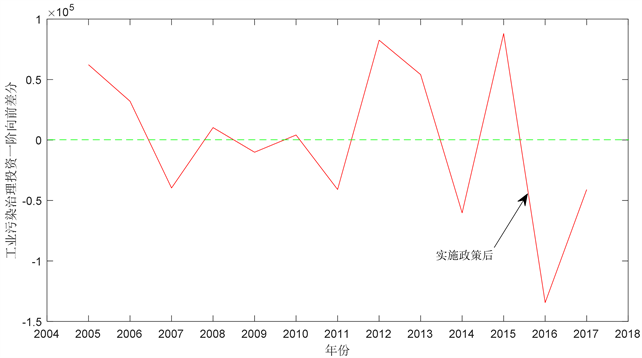Figure 4. First order difference time sequence diagram of industrial pollution control investment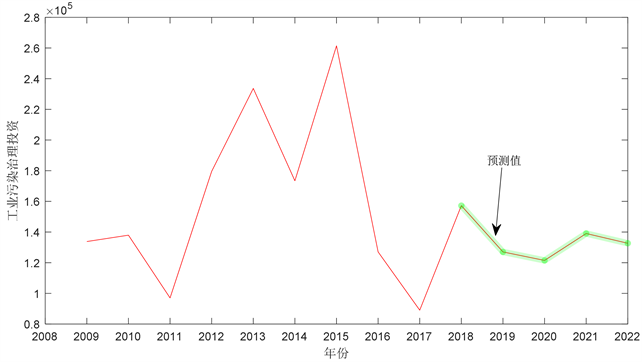Figure 5. Time sequence chart of investment prediction for industrial pollution control

5. 对促进湖南省经济发展和提高经济活力的建议

1) 结合本省实际情况，大力推进长株潭经济一体化，并以此为契机，提高其周边区域的城市化水平，进而提高湖南省的城市化水平。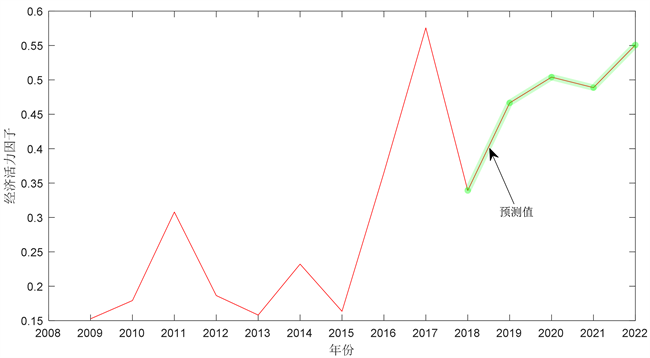Figure 6. Time sequence chart of economic vitality factor prediction

2) 大力兴办高科技企业，因地制宜发展区域特色产业，增强全省的企业质量，并通过技术创新提高其竞争力，从而更实际地让企业的发展带动GDP的增长。

3) 注重发展教育和引进人才，提高全省人民的思想道德素质，提高全省的人力资源水平。

4) 鼓励移动投资与引进外资企业，加强对外交流与合作，减少固定资产投资，增加对教育等对经济活力具有突出贡献的领域的投资，注重挖掘投资潜力。

5) 注重基础实施建设，改善基层民生，增强人民的幸福感。

6) 把握机遇，迎接挑战。面对中央出台的新政策，应当积极响应，并结合本省具体实际进行适当的调整与彻底的落实。

7) 积极贯彻经济可持续发展和绿色发展战略。调整产业结构，着力发展第二、第三产业和新兴产业，坚决淘汰或者转移高污染产业和高耗能产业，让经济发展与节约资源、保护环境相协调。

6. 结束语

The Prediction of Economic Vitality in Hunan Province and Its Influencing Factors[J]. 统计学与应用, 2020, 09(01): 81-89. https://doi.org/10.12677/SA.2020.91010

1. 1. 逯进, 王晓飞. 固定资产投资、老龄化与经济活力——基于省域视角研究[J]. 人口学刊, 2019, 41(5): 57-71.

2. 2. 程莉, 滕祥河, 文传浩. 人口城镇化质量对经济增长影响的实证分析[J]. 统计与决策, 2017(2): 136-139.

3. 3. 钱诚超. 多元化统计的湖北省企业数量与经济增长关系的实证分析[J]. 商场现代化, 2018(2): 167-169.

4. 4. 何汝群. 珠江–西江经济带城市经济活力评价研究[D]: [硕士学位论文]. 广西师范大学, 2019.

5. 5. 司守奎, 孙兆亮. 数学建模算法与应用[M]. 第2版. 北京: 国防工业出版社, 2019, 167-306.